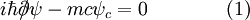# Majorana Equationcan someone please explain this realistic wave equation
i'm new with spinors :)

Last edited by a moderator:

I think you mean "relativistic wave equation" also called the "Dirac equation". the slashed $$\partial$$ is Feynman's short form for $$\gamma^\mu\partial_\mu$$ where $$\gamma^\mu$$'s are the Dirac matrices (4x4) and $$\mu=0,1,2,3$$. In essence, it is just an equation saying that $$E^2-p^2=m^2$$ for a spin-1/2 particle with wave function $$\psi$$. $$\psi$$ is a 4-component object.
The wikipedia article where you got the image from explains that this is a similar equation to the Dirac equation, but, however, with the difference that it contains the spinor and its conjugate in the same equation (there are two separate Dirac equations for the spinor and its congujate). This is due to the fact that the Majorana spinor has its particle equal to its antiparticle, $\psi = \mathcal{C}\psi\mathcal{C}^{-1}$ with $\mathcal{C}$ charge conjugation. This condition implies $\psi = - i \gamma^2 \psi^{\ast}$ as you can verify in Peskin and Schroeder eq. (3.145) ($\gamma^2$ the second gamma matrix and $\psi^{\ast}$ the complex conjugate). This is sometimes called Majorana condition. The properties of this spinors as well as the Lagrangian that lead to that equation are explained in exercise 3.13 of Peskin and Schroeder. Majorana spinors are postulated to explain neutrino physics.